# Texas Go Math Grade 8 Lesson 12.4 Answer Key Algebraic Representations of Transformations

Refer to our Texas Go Math Grade 8 Answer Key Pdf to score good marks in the exams. Test yourself by practicing the problems from Texas Go Math Grade 8 Lesson 12.4 Answer Key Algebraic Representations of Transformations.

## Texas Go Math Grade 8 Lesson 12.4 Answer Key Algebraic Representations of Transformations

Question 1.
A rectangle has vertices at (0, -2), (0, 3), (3, -2), and (3, 3). What are the coordinates of the vertices of the image after the translation (x, y) → (x – 6, y – 3)? Describe the translation.
Coordinates of the vertices of the image after the translation are:
A'(-6, -5);
B'(-6, 0);
C'(-3, -5);
D'(-3, 0).
The translation is 6 units to the left and 3 units down.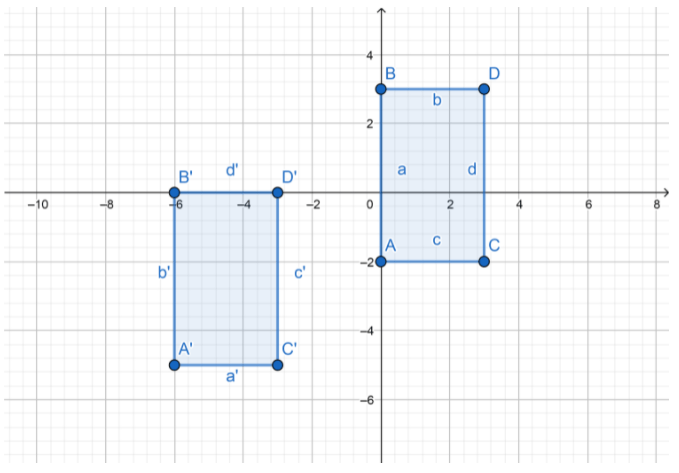Question 2.
Triangle ABC has vertices A(-2, 6), 8(0, 5), and C(3, -1). Find the vertices of triangle A’B’C’ after a reflection across the x-axis.
The vertices of triangle A’B’C’ after a reflection across the x-axis are:
A'(-2, -6);
B'(0, -5);
C'(3, 1).Reflect

Question 3.
Communicate Mathematical Ideas How would you find the vertices of an image if a figure were rotated 270° clockwise? Explain.
If a figure is rotated 270° clockwise, it’s the same like the figure is rotated 90° counterclockwise. Multiply each y- coordinate by 1, then switch the x- and y-coordinates: (x, y) will be (-y, x).

Question 4.
A triangle has vertices at j(-2, -4), K(1, 5), and L(2, 2). What are the coordinates of the vertices of the image after the triangle is rotated 90° counterclockwise?
Step 1: Apply the rule to find the vertices of the image. Multiply each y-coordinate by 1, then switch x- and y coordinates. (x, y) will be (-y, x).
For J(-2, -4) the image is J'(4, -2);
For K(1, 5) the image is K'(-5, 1);
For L(2, 2) the image is L'(-2, 2).

Step 2: Graph the triangle and its image.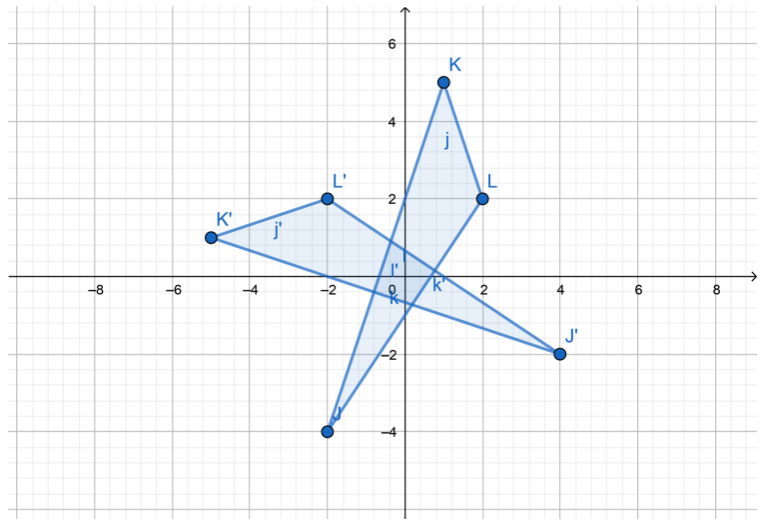Question 1.
Triangle XYZ has vertices X(-3, -2), Y(-1, 0), and Z(1, -6). Find the vertices of triangle X’Y’Z’ after a translation of 6 units to the right. Then graph the triangle and its image. (Example 1)Step 1: Apply the rule to find the vertices of the image. Add 6 to the x-coordinate (x, y) will be (x + 6, y).
For X(-3, -2) the image is X'(3, -2);
For Y(-1, 0) the image is Y'(5, 0);
For Z(1, -6) the image is Z'(7, -6).

Step 2: Graph the triangle and its image.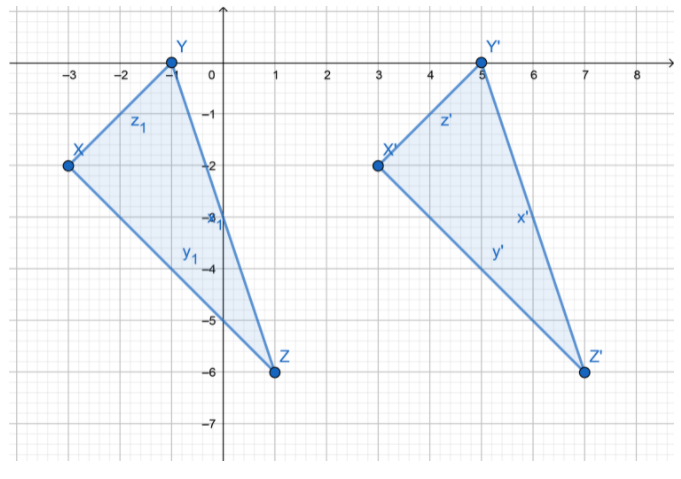Question 2.
Describe what happens to the x- and y-coordinates after a point is reflected across the x-axis. (Example 2)
After the point is reflected across the x-axis, the x coordinate does not change and the y coordinate is multiplying by 1.
(x, y) will be (x, -y)

Question 3.
Use the rule (x, y) → (y, -x) to graph the image of the triangle at right. Then describe the transformation. (Example 3)
Step 1 Apply the rule to find vertices of the image.
A(-4, 2) → A'(2, 4)
B(2, 3) → B'(3, -2)
C(-3, 4) → C'(4, 3)
This means that transformation is rotation 90° clockwise.

Step 2: Graph the triangle and its imageEssential Question Check-In

Question 4.
How do the x- and y-coordinates change when a figure is translated right a units and down b units?The x-coordinates increase a units, and y-coordinates decrease b units.

Write an algebraic rule to describe each transformation. Then describe the transformation.

Question 5.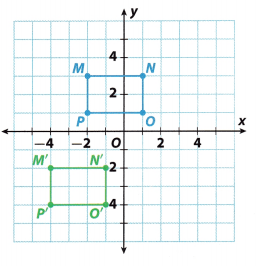The point (x, y) changes to (x – 2, y – 5). This is an algebraic rule.
The transformation is a translation of 2 units left and 5 units down.

Question 6.The point (x, y) changes to (-x, -y). This is an algebraic rule.
The transformation is a rotation for 180°.

Question 7.
Triangle XYZ has vertices X(6, -2.3), Y(7.5, 5), and Z(8, 4). When translated, X’ has coordinates (2.8, -1.3). Write a rule to describe this transformation. Then find the coordinates of Y’ and Z’.
X(6, -2.3) → X'(2.8, -1.3)
Find how changes coordinate x.
6 – a = 2.8
a = 6 – 2.8
a = 3.2
And how changes coordinate y.
-2.3 + b = – 1.3
b = 1.3 – (-2.3)
b = -1.2 + 2.3
b = 1
The rule is (x, y) → (x – a, y + b) where a = 3.2 and b = 1.
Apply the rule to find points Y’ and Z’.
Y(7.5, 5) → Y'(7.5 – 3.2, 5 + 1) So, Y’ has coordinates (4.3, 6).
Z(8, 4) → Z'(8 – 3.2, 4 + 1). So, Z’ has coordinates (4.8, 5).

Question 8.
Point L has coordinates (3, -5). The coordinates of point L’ after a reflection are (-3, -5). Without graphing, tell which axis point L was reflected across. Explain your answer.
The reflection is across the y-axis, because coordinate x of point L changed sign.

Question 9.
Use the rule (x, y) → (x – 2, y – 4) to graph the image of the rectangle. Then describe the transformation.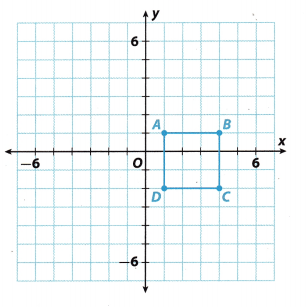Apply the ruLe (x, y) → (x – 2, y – 4) to find the vertices of the image A’B’C’D’.
A(1, 1) → A'(-1, -3)
B(4, 1) → B'(2, -3)
C(4, -2) → C'(2, -6)
D(1, -2) → D'(-1, -6)
The transformation is a translation 2 units left and 4 units down.
Graph the rectangle A’B’C’D’.Question 10.
Parallelogram ABCD has vertices A(-2, -5$$\frac{1}{2}$$), 6(-4, -5$$\frac{1}{2}$$), C(-3, -2), and D(- 1, -2). Find the vertices of parallelogram A’B’C’D’ after a translation of 2$$\frac{1}{2}$$ units down.
The transformation is a translation of 2$$\frac{1}{2}$$ down.
This means that y coordinate change.
Subtract 2$$\frac{1}{2}$$ from the y coordinate.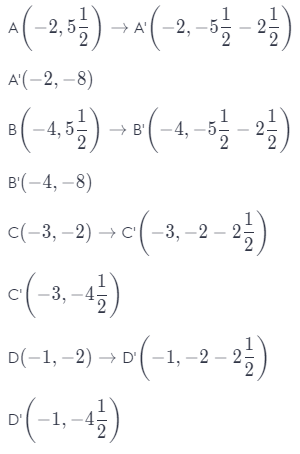Question 11.
Alexandra drew the logo shown on half-inch graph paper. Write a rule that describes the translation Alexandra used to create the shadow on the letter A.The translation is 1 unit right and 0.5 unit down.
So, add 1 to the x coordinate and subtract 0.5 from the y coordinate.
The rule is:
(x, y) → (x + 1, y – 0.5)
One unit is one half inch.
So, the rule also can be add 0.5 inch (1 unit) to the x coordinate and subtract 0.25 inch (0.5 unit) from the y coordinate.
(x, y) → (x + 0.5 in, y – 0.25 in)

Question 12.
Kite KLMN has vertices at K(1, 3), L(2, 4), M(3, 3), and N(2, 0). After the kite is rotated, K’ has coordinates (-3, 1). Describe the rotation, and include a rule in your description. Then find the coordinates of L’, M’, and N’.
K(1, 3) → K'( 3, 1)
From the point K’ we can see that the rule is:
(x, y) → (y, x)
and this is a rule for the rotation 90° counterclockwise.
Apply it to all points.
L(2, 4) → L'(-4, 2)
M(3, 3) → M'(-3, 3)
N(2, 0) → N'(0, 2)

H.O.T. Focus on Higher Order Thinking

Question 13.
Make a Conjecture Graph the triangle with vertices (-3, 4), (3, 4), and (-5, -5). Use the transformation (y, x) to graph its image.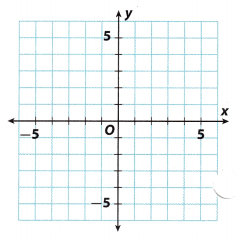a. Which vertex of the image has the same coordinates as a vertex of the original figure? Explain why this is true.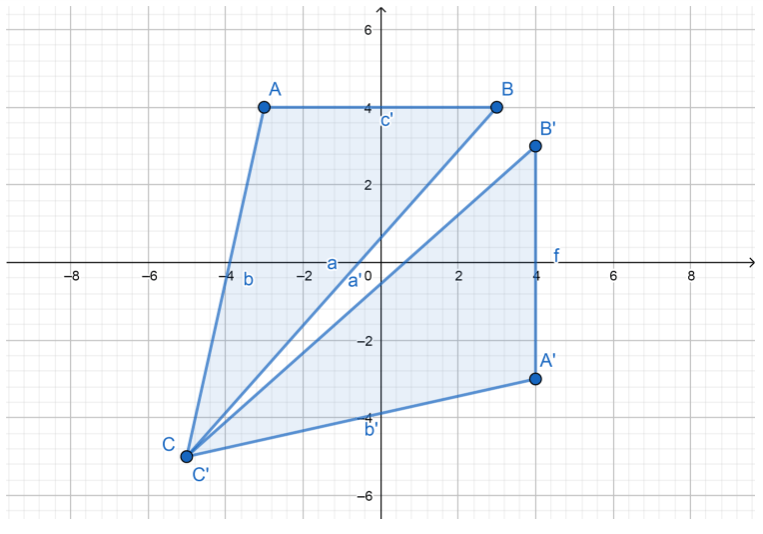The point C’ has the same coordinates as a vertex C, because the transformation is:
(x, y) → (y, x) and vertex C has coordinates where x = y. If we replace them, we will get the same point C (-5, -5)

b. What is the equation of a line through the origin and this point?
First find coefficient k, if:The equation of a line through two points (in this case O and C’) is:
y – y1 = k(x – x1)
y – 0 = 1 (x – 0)
y = x

c. Describe the transformation of the triangle.
This transformation is a reflection across the line y = x, because the triangle ABC and A’B’C’ are the same distance from the line y = x, which is a line of reflection.

Question 14.
Critical Thinking Mitchell says the point (0, 0) does not change when reflected across the x- or y-axis or when rotated about the origin. Do you agree with Mitchell? Explain why or why not.
Yes, applying the algebraic rules for the reflection and the rotation on the point O(0, 0), we will get the same point O.
For example reflection across the x-axis:
(x, y) → (x, -y)
(0, 0) → (0, 0)
This also applies to other transformations.

Question 15.
Analyze Relationships Triangle ABC with vertices A(-2, -2), B(-3, 1), and C(1, 1) is translated by (x, y) → (x – 1, y + 3). Then the image, triangle A’B’C’, is translated by (x, y) → (x + 4, y – 1), resulting in A”B”C”.
a. Find the coordinates for the vertices of triangle A”B”C”.
First find the coordinates for the vertices B’ and C’.
Apply the rule (x, y) → (x – 1, y + 3).
A(- 2, 2) → A'(-3, 1)
B(-3, 1) → B'(-4, 4)
C(1, 1) → C'(0, 4)
Then apply the rule (x, y) → (x + 4, y – 1) on the coordinates of vertices A’, B’ and C’ to find the coordinates of vertices A”, B” and C”.
A'(- 3, 1) → A”(1, 0)
B'(-4, 4) → B”(0, 3)
C'(0, 4) → C”(4, 3)

b. Write a rule for one translation that maps triangle ABC to triangle A”B”C”.